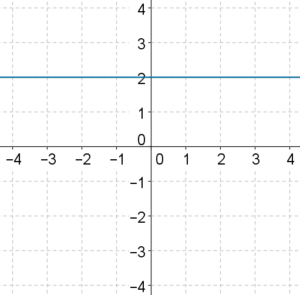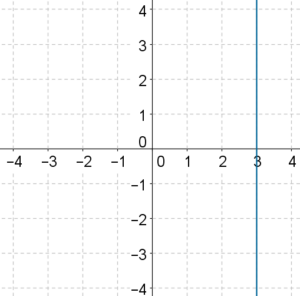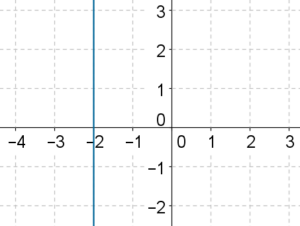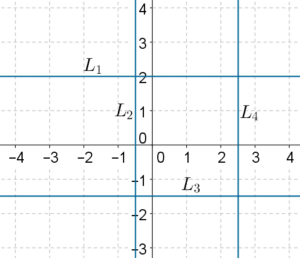# Lines Parallel to Axes

Go back to  'Two Variable Linear Equations'

Consider the equation $$y = 2$$, or $$y - 2 = 0$$. This is an equation with a single variable y. However, we can think of it as a two-variable linear equation in which the coefficient of x is 0:

$\left( 0 \right)x + \left( 1 \right)y + \left( { - 2} \right) = 0$

No matter what value we substitute for x, the value of y will always come out to be 0. Thus, all solutions of this two-variable linear equation are of the form $$\left( {k,2} \right)$$, where k is some real number.

What will the graph of this linear equation look like? Well, we plot all points in the plane for which the y-coordinate is 2:This is a line parallel to the x-axis. Thus, an equation of the form $$y = a$$ represents a straight line parallel to the x-axis and intersecting the y-axis at $$\left( {0,a} \right)$$.

Now, consider the equation $$x = 3$$. This can also be written as a two-variable linear equation, as follows:

$\left( 1 \right)x + \left( 0 \right)y + \left( { - 3} \right) = 0$

Clearly, no matter what value we substitute for y, the value of x will always come out to be 3. Thus, the solutions of this equation are all of the form $$\left( {3,k} \right)$$, where k is some real number. The graph of this equation will consist of all points whose x-coordinate is 3, that is, a line parallel to the ­y-axis, and passing through $$\left( {3,0} \right)$$:In general, an equation of the form $$x = a$$ represents a straight line parallel to the y-axis and intersecting the x-axis at $$\left( {a,0} \right)$$.

Example 1: What does the equation $$2x + 3 = - 1$$ represent when considered as a linear equation in

I. one variable?

II. two variables?

Solution: I. When considered as a linear equation in one variable, this equation simply gives us one (unique) solution for x, which is $$x = - 2$$. We can represent the solution on a (one-dimensional) number line as a single point:II. However, when considered as a linear equation in two-variables, this represents a line parallel to the y-axis, as shown below:Example 2: The following figure shows four lines, each of which is parallel to one of the two axes.Determine the equation of each line.

Solution: $${L_1}$$ is parallel to the x-axis and passes through $$\left( {0,2} \right)$$. Thus, the equation of $${L_1}$$ will be $$y = 2$$.

$${L_2}$$ is parallel to the y-axis and passes through $$\left( { - \frac{1}{2},0} \right)$$. The equation of $${L_2}$$ will be $$x = - \frac{1}{2}$$.

Similarly, the equation of $${L_3}$$ will be $$y = - \frac{3}{2}$$ and that of $${L_4}$$ will be $$x = \frac{5}{2}$$.

Linear Equations
Linear Equations
grade 9 | Questions Set 2
Linear Equations